# Load Flow or Power Flow Analysis

It is the computational procedure (numerical algorithms) required to determine the steady state operating characteristics of a power system network from the given line data and bus data.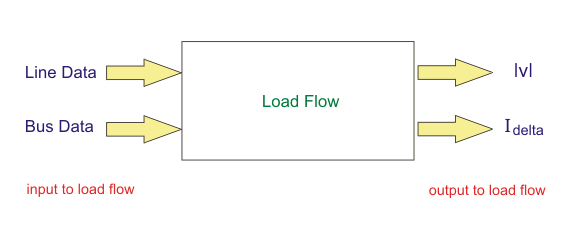1. Load flow study is the steady state analysis of power system network.
3. Load flow solves a set of simultaneous non linear algebraic power equations for the two unknown variables (|V| and ∠δ ) at each node in a system.
4. To solve non linear algebraic equations it is important to have fast, efficient and accurate numerical algorithms.
5. The output of the load flow analysis is the voltage and phase angle, real and reactive power (both sides in each line), line losses and slack bus power.

The study of load flow involves the following three steps:

1. Modeling of power system components and network.
2. Development of load flow equations.
3. Solving the load flow equations using numerical techniques.

### Modeling of Power System Components

Generator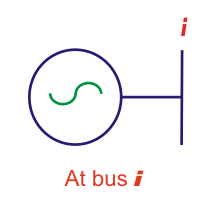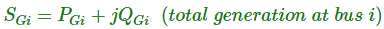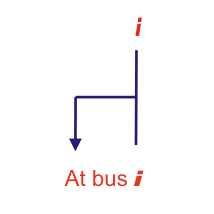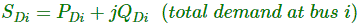Transmission Line
A Transmission line is represented as a nominal π model.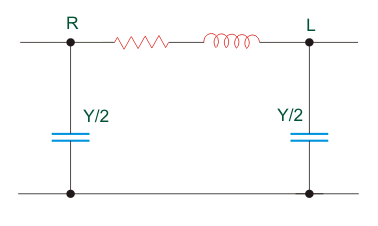Where, R + jX is the line impedance and Y/2 is called the half line charging admittance.

Off Nominal Tap Changing Transformer
For a nominal transformer the relation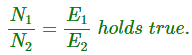But for an off nominal transformer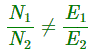Thus for an off nominal transformer we define the transformation ratio (a) as follows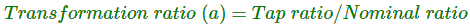Now we would like to represent an off nominal transformer in a line by an equivalent model.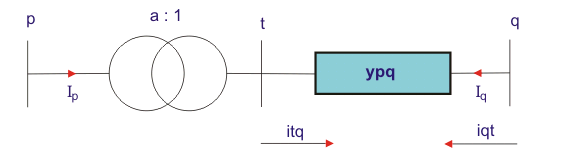Fig 2: Line Containing an Off Nominal Transformer
We want to convert the above into an equivalent π model between bus p and q.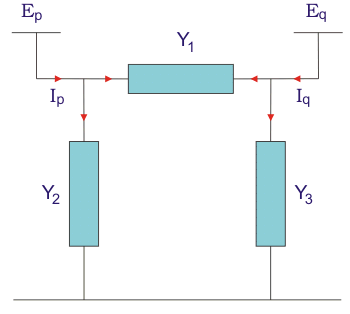Fig 3: Equivalent π Model of Line

Our aim is to find these values of admittances Y1, Y2 and Y3 so that fig2 can be represented by fig 3
From Fig 2 we have,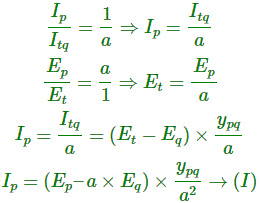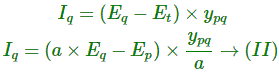Now consider Fig 3, from fig3 we have,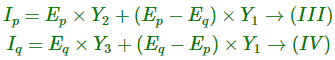From eqn I and III on comparing the coefficients of Ep and Eq we get,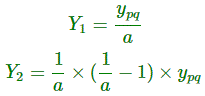Similarly from equation II and IV we have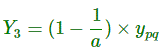Some useful observations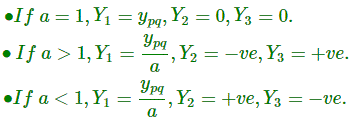From above analysis we see that Y2, Y3 values can either be positive or negative depending on the value of transformation ratio.Good question!
Y = – ve implies absorption of reactive power i.e it is behaving as an inductor.
Y = + ve implies generation of reactive power i.e it is behaving as a capacitor.
Modeling of a Network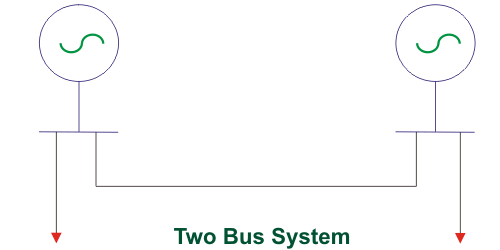Consider the two bus system as shown in figure above.
Power generated at bus i is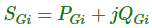Power demand at bus i is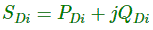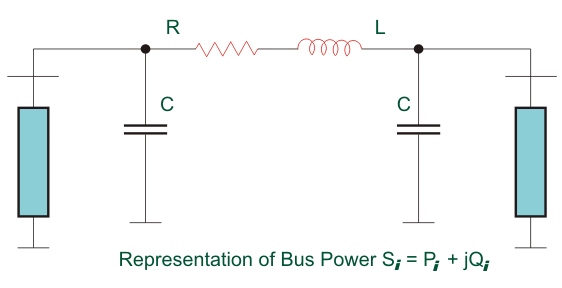Therefore we define the net power injected at bus i as follows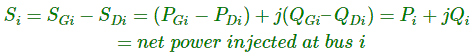Want To Learn Faster? 🎓
Get electrical articles delivered to your inbox every week.
No credit card required—it’s 100% free.